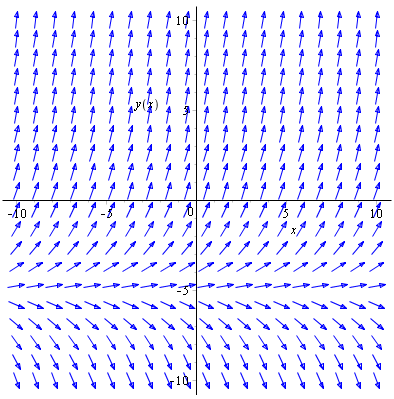First order differential equations

Introduction

Any relation between $y$, $\frac{dy}{dx}$, $\frac{d^{2}y}{dx^{2}}$, ... is called a differential equation.

If the highest order derivative in an equation is $\frac{d^{n}y}{dx^{n}}$ then the differential equation is order $n$.

When solving a differential equation we aim to find the base relationship between $y$ and $x$ in as simple terms as possible.

Separation of variables

In C3 you learned that differential equations can sometimes be separated so that the solution can be found by integrating

$$\frac{dy}{dx} = \frac{f(x)}{g(y)}$$

$$\Rightarrow \int g(y) \mathop{dy} = \int f(x) \mathop{dx}$$

This yields an integral on either side with a constant of integration. The constants of integration on either side can be merged into a single arbitrary constant.

The presence of an arbitrary constant means you have gained a general solution. In particular situations you may already know something about the solution function. Maybe you know that at $x=0$, $y=0$. That is a boundary condition which you sub in to the general solution to find the particular solution.

Example

Q) Solve $\frac{dy}{dx} = \frac{y-1}{x}$, given that $y=0$ when $x=1$.

A) By rearranging we can separate variables

$$\frac{dy}{dx} = \frac{y-1}{x}$$

$$\frac{1}{y-1}\frac{dy}{dx} = \frac{1}{x}$$

$$\Rightarrow \int \frac{1}{y-1} \mathop{dy} = \int \frac{1}{x} \mathop{dx}$$

So we come up with a single arbitrary constant of integration $C$

$$\ln\left|y-1\right| = \ln\left|x\right| + C$$

$$e^{\ln\left|y-1\right|} = e^{\ln\left|x\right| + C}$$

Let $a = e^{C}$, so

$$y-1 = ax$$

$$\Rightarrow y = ax+1$$

That is the general solution. But we were told the boundary condition $y\left(1\right)=0$ so

$$0 = a +1 \Rightarrow a = -1$$

Therefore the particular solution is $y = 1-x$.

Family of solution curves

A consequence of the arbitrary constant in general solutions is that you can plot a family of solutions curves for many values of the arbitrary constant.

In the last example the general solution was $y = ax+1$. So the family of solutions curves is a load of functions all with different values of the constant $a$. Looking at the general solution, all the curves will be straight lines passing through $(0,1)$. The only thing to vary will be the gradient of the straight lines.

Here's what they look like. The highlighted line is the particular solution $y = 1-x$ arising from the boundary condition $y\left(1\right)=0$.Each arrow points in the direction of the gradient $\frac{dy}{dx}$ at that point in the 2D plane. The original differential equation $\frac{dy}{dx} = \frac{y-1}{x}$ shows that $\frac{dy}{dx}$ varies with both $x$ and $y$, and so the gradient is different depending on where you are in the 2D plane.

Example

Q) Find the general solution of the differential equation $\frac{dy}{dx} = \frac{y+5}{2}$ and sketch the family of solutions curves.

A) Rearranging and integrating

$$\frac{dy}{dx} = \frac{y+5}{2}$$

$$\int \frac{2}{y+5} \mathop{dy} = \int dx$$

$$\Rightarrow 2\ln\left|y+5 \right| = x + C$$

$$\ln\left|y+5 \right| = \frac{x + C}{2}$$

$$\Rightarrow y+5 = e^{\frac{x + C}{2}}$$

Let $a = e^{\frac{C}{2}}$, so

$$y = ae^{\frac{x}{2}}-5$$

And the family of solutions curvesThe original differential equation $\frac{dy}{dx} = \frac{y+5}{2}$ shows that $\frac{dy}{dx}$ only varies with $y$ and not $x$. That means that the gradient at each point only changes if you go upwards and downwards. The gradients stay constant as you move horizontally from point to point.

First order exact differential equations

Take the differential equation

$$y + x\frac{dy}{dx} = \sin x$$

The variables can't be separated, but notice that the left hand side is equal to $\frac{d}{dx}(xy)$ from the product rule. Then the differential equation becomes

$$\frac{d}{dx}(xy) = \sin x$$

Integrate both sides with respect to $x$ to get the general solution

$$xy = -\cos x + C$$

Differential equations of this form where one side is the exact derivative of a product, and the other can be integrated with respect to the independent variable ($x$ in this case) is an first order exact differential equation.

First order linear differential equations

A first order linear differential equation takes the form

$$\frac{dy}{dx} + Py = Q$$

where $P$ and $Q$ can be functions of $x$ or constants. We can make the differential equation exact by multiplying both sides by an integrating factor, a function of $x$ gained by using $P$.

Specifically the integrating factor is equal to

$$e^{\int P \mathop{dx}}$$

Suppose there exists a function $f(x)$ such that the linear differential equation $$\frac{dy}{dx} + Py = Q$$ where $P$ and $Q$ are either functions of $x$ or constants, is made an exact differential equation by multiplying through by $f(x)$. In other words $$f(x) \frac{dy}{dx} + f(x)Py \equiv \frac{d}{dx}\left[f(x)y\right]$$ But by the product rule $$\frac{d}{dx}\left[f(x)y\right] = f(x) \frac{dy}{dx} + f'(x)y$$ By comparing the two, $$f(x)P = f'(x)$$ Divide both sides by $f(x)$ and integrate with respect to $x$ $$\int P \mathop{dx} = \int \frac{f'(x)}{f(x)}\mathop{dx} = \ln\left(f(x)\right)$$ Therefore $$f(x) = e^{\int P \mathop{dx}}$$

Example

Q) Find the general solution of $\frac{dy}{dx} + \frac{y}{x} = 2$.

A) $P(x) = \frac{1}{x}$ and $Q(x) = 2$. Therefore the integrating factor is

$$e^{\int \frac{1}{x} \mathop{dx}} = e^{\ln x } = x$$

Multiply both sides by the integrating factor

$$\frac{dy}{dx}x + y = 2x$$

The left hand side is equal to $\frac{d}{dx}\left(xy\right)$, so integrate both sides with respect to $x$

$$\frac{d}{dx}\left(xy\right) = 2x$$

$$\Rightarrow xy = \int 2x \mathop{dx} = x^{2} + C$$

Therefore the general solution is

$$xy = x^{2} + C$$

Where $C$ is an arbitrary constant.